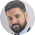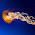Data Interpretation Practice Questions - Missing Figures Tables

In a series to provide practice question sets for Data Interpretation section, Today I am providing two practice sets.

Study the given table carefully to answer the following questions.

Field Name
Shape
Side (in m)
Base (in m)
Height (in m)
Cost of flooring (in Rs. per sq. metre)
Cost of fencing (in Rs. per m)
A
Triangle

16
12

50
20
B
Rectangle
10 × 20

30
15
C
Square
15

40
18
D
Parallelogram

20
12

60
25
E
Circle

10
45
22

1) What is the cost of flooring of A?
a) Rs.4000                   b) Rs.4600                   c) Rs.4800
d) Rs.5000                   e) Rs.4400

2) What is the difference between the cost of fencing of C and that of B?
a) Rs.180                     b) Rs.120                     c) Rs.240
d) Rs.360                     e) Rs.480

3) What is the ratio of the cost of flooring to that of fencing of field D?
a) 4 : 1                         b) 6 : 1                         c) 8 : 1
d) 9 : 1                         e) 5 : 1

4) The cost of fencing of field E is approximately what percent of the cost of flooring of field C?
a) 10.5%                      b) 19.46%                    c) 18.71%
d) 15.36%                    e) 13.82%

5) The cost of fencing of field C is what percent of the cost of fencing of field D?
a) 87.54%                    b) 67.5%                      c) 72.13%
d) 54.36%                    e) 46.5%

Study the given chart carefully and answer the following questions.

Train A

Station
Arrival time
Departure time
Distance from origin (in km)
Number of passengers boarding at each station
Fare (in Rs.)
Starting
5:00 pm
--
400
--
6:30 pm
6:35 pm
100
100
50
Bharuch
8:50 pm
9:00 pm
250
90
120
Mumbai
4:00 am
4:10 am
800
300
400
Pune
7:30 am
7:45 am
1050
150
500
Solapur
10:20 am
Terminates
1280
--
620

Train B

Station
Arrival time
Departure time
Distance from origin
Number of passengers boarding at each station
Fare (in Rs.)
Solapur
Starting
6:00 pm
--
300
--
Pune
7:40 pm
7:45 pm
230
150
120
Mumbai
9:30 pm
9:35 pm
480
270
220
Bharuch
5:40 am
5:55 am
1030
50
500
9:00 am
9:10 am
1180
100
570
12:00 noon
Terminates
1280
--
620

6) The number of passengers boarding Train A at Vadodara is what percent of the number of passengers boarding Train B at Mumbai?
a) 37.03%                    b) 47.03%                                c) 27.03%
d) 47.30%                    e) None of these

7) What is the difference between the speed of Train A and that of Train B?
a) 2.73 kmph               b) 1.97 kmph                           c) 3.6 kmph
d) 2.62 kmph               e) 3.9 kmph

8) What is the ratio of the total passengers of Train A to that of Train B?
a) 102 : 79                   b) 104 : 87                               c) 103 : 87
d) 110 : 79                   e) 113 : 87

9) The total income of Train A is what percent of the total income of Train B?
a) 180%                       b) 159.51%                              c) 123.29%
d) 125%                       e) 127.64%

10) If the average speed of Train A increases by 10% then when will it reach to its destination?
a) 7:45 am                   b) 9:45 am                               c) 8:45 am
d) 10:45 am                 e) 11:45 am

Solutions

1. Option C
A is a triangle
So, area of A = 1/2 × 16 × 12 = 96 sqm
So, cost of flooring of A = 96 × 50 = Rs.4800

2. Option A
Perimeter of B = 2 (10 + 20) = 60 m
So, cost of fencing of B = 60 × 15 = 900
Perimeter of C = 4 × 15 = 60 m
So, cost of fencing of C = 60 × 18 = Rs.1080
So, required difference = 1080 ⎯ 900 = Rs.180

3. Option D
Area of D = Base × Height
= 20 × 12 = 240 mtr sq
So, cost of flooring of D= 240 × 60 = Rs.14400
Perimeter of D = 2 (20 + 12) = 64 m
So, cost of fencing of D = 64 × 25 = Rs.1600
So, required ratio = 14400 : 1600 = 9 : 1

4. Option D
Perimeter of E = 2πr = 2 × 22/7 × 10 = 440/7 m
Cost of fencing of E = 440/7 × 22 = Rs.1382.85
Area of C = 15 * 15= 225 mtr square
So, cost of flooring of C = 225 × 40 = Rs.9000
So, required % = 1382.85 x 100 / 9000
= 15.36% of flooring cost of C.
5. Option B
Fencing cost of C = Rs.1080
Fencing cost of D = Rs.1600
Required % = 1080/1600 × 100 = 67.5%

6. Option A
Required percentage = 100/270 × 100 = 37.03%

7. Option A
Speed of Train A = 1280 / 10:20 am – 5:00 pm
= 1280 / 17 hours 20 minutes
= 1280 x 3 / 52 = 73.84 kmph
Speed of train B = 1280 / 12:00 noon ⎯ 6:00 pm
= 1280/18 hours = 71.11 kmph
So, difference between the speed of train A and train B = 73.84 ⎯ 71.11 = 2.73 kmph

8. Option B
Total passengers in train A = 400 + 100 + 90 + 300 + 150 = 1040
Total passengers in train B = 300 + 150 + 270 + 50 + 100 = 870
So, required ratio = 1040 : 870 = 104 : 87

9. Option E
Total income of train A = (400 × 50) + (500 × 70) + (590 × 280) + (890 × 100) + (1040 × 120) = Rs.434000
Total income of train B = (300 × 120) + (450 × 100) + (620 × 280) + (670 × 70) + (770 × 50) = Rs.340000
So, required % = 434000 x 100 / 340000
= 127.64% of the total income of train B.

10. Option C
If the average speed of train A increases by 10%
then its new speed = 73.84 × 110/100
= 81.22 kmph
Time taken by train A during the journey = 1280/81.22 = 15.75 hours = 15 hours 45 minutes
The time when the train will reach its destination = 5 pm + 15 hours 45 minutes = 8:45 amWhat's trending in BankExamsToday

Smart Prep Kit for Banking Exams by Ramandeep Singh - Download here

Join 40,000+ readers and get free notes in your email1.how will i get the data interpretation work book after making payment through my debit card

1.You will get soft copy in your mail.

Hard copy of book will be available within 10 days.

2.Sir apne bola tha 8 rti ka data aapke paas hai please share kariye ! jaan ataki hui hai reserve list ke chakkar mai

1.Actually there are many secret groups on Facebook, these groups are taking initiatives to fight for candidate's rights.

3.Please explain Q.no. 8 ..
why are you counting passenger for each station while they already paid ticket amount on the boarding station.
total income of train A should be =(400X50)+(100X70)+(90X280) +(300X100)+(150X120)
please correct me if i am wrong..

4.Dear sir,
Pls upload recent held IBPS RRB exam questions and answer for English language and computer papers (07 Sept 2014 )when you upload in bank exam portal ,pl kindly replay & help us

1.I will do that soon

5.Paschima gaa ra gotte paikhana pakhere aachi

6.while solving question no. 7 why we are not subtracting the halt time from total time span ...sir cn u plz explain

7.The solution of Ques No 3 is incorrect. Kindly review.. Perimeter of Parallelogram = 2(a+b) where a and b are the adjoining sides of the parallelogram. But here the height has been taken as the other side and answer has been derived.

Thanks for commenting. Follow us on Telegram. Search BankExamsToday